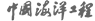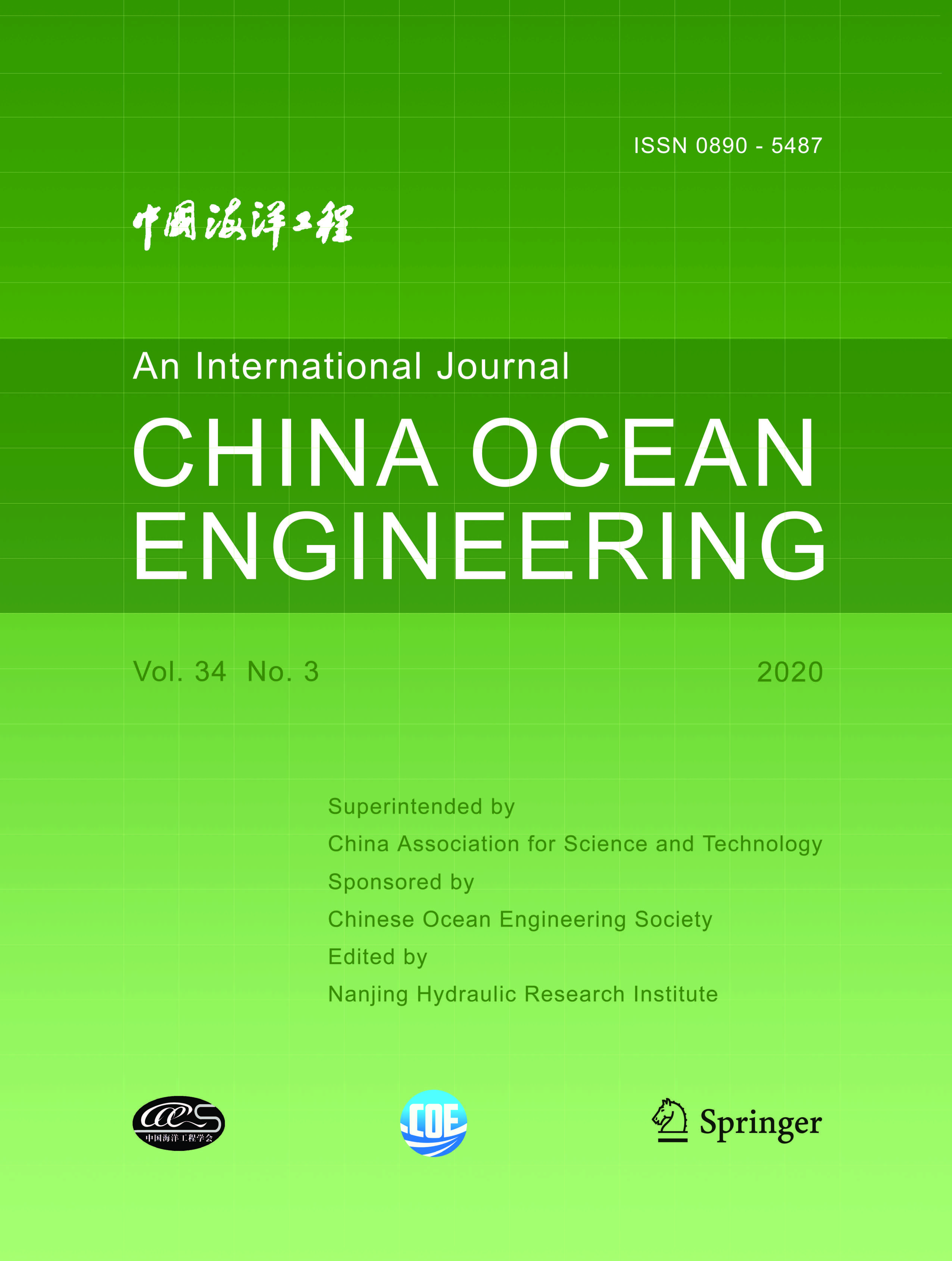ISSN  0890-5487 CN 32-1441/P

## 2001 Vol.(2)

Display Mode：          |

2001, (2): -.
[Abstract](378)
Abstract:
Based on the fluid motion equations, the physical meaning of eddy viscosity coefficient and the rationality of the Boussinesq hypothesis are discussed in this paper. The effect of the coefficient on numerical stability is analyzed briefly. A semi-enclosed rectangular sea area, with an orthogonal spur dike, is applied in a 2-D numerical model to study the effect of horizontal eddy viscosity coefficient (AH). The computed result shows that AH has little influence on the tidal level and averaged flow velocity, but has obvious influence on the intensity and the range of return flow around near the spur dike. Correspondingly, a wind-driven current pool and an annular current are applied in a 3-D numerical model respectively to study the effect of vertical eddy viscosity coefficient (Av). The computed result shows that the absolute value of Av is inversely proportional to that of horizontal velocity, and the vertical gradient value of Av determines the vertical distribution of horizontal velocity. The distribution form of Av is theoretically recommended as a parabolic type, of which the maximum value appears at 0.5 H.
2001, (2): -.
[Abstract](346)
Abstract:
The possible motions of a caisson breakwater under dynamic load excitation include vibrating, vibrating-sliding and vibrating-rocking motions. The models of vibrating motion and vibrating-sliding motion have been proposed in an early paper. In this paper, a model of vibrating-rocking motion of caisson breakwaters under breaking wave impact is presented, which can be used to simulate the histories of vibrating-rocking motion of caisson breakwaters. The effect of rocking motion on the displacement, rotation, sliding force and overturning moment of breakwaters is investigated. In case the overturning moment exceeds the stability moment of a caisson, the caisson may only rock. The caisson overturns only in case the rocking angle exceeds the critical angle. It is shown that the sliding force and overturning moment of breakwaters can be reduced effectively due to the rocking motion. It is proposed that some rocking motion should be allowed in breakwater design.
2001, (2): -.
[Abstract](315)
Abstract:
The "surface roller" to simulate wave energy dissipation of wave breaking is introduced into the random wave model based on approximate parabolic mild slope equation in this paper to simulate the random wave transportation including diffraction, refraction and breaking in nearshore areas. The roller breaking random wave higher-order approximate parabolic equation model has been verified by the existing experimental data for a plane slope beach and a circular shoal, and the numerical results of random wave breaking model agree with the experimental data very well. This model can be applied to calculate random wave propagation from deep to shallow water in large areas near the shore over natu ral topography.
2001, (2): -.
[Abstract](336)
Abstract:
2001, (2): -.
[Abstract](336)
Abstract:
A Three-Scale Fuzzy Analytical Hierarchy Process (T-FAHP) is proposed by introducing the Three-Scale Analytical Hierarchy Process (T-AHP) and the trapezoid fuzzy number. A multi-objective optimization model based on the T-FAHP is presented subsequently, in which many factors influencing the lectotype of offshore platform are taken into account synthetically, such as the original investment, the maintenance cost, the ability of resisting fatigue and corrosion, the construction period, the threat to the environment, and so on. With this method, the experts can give the relatively precise ranking weight of each index and at the same time the requirement of consistence checking can be met. The result of a calculation example shows that the T-FAHP is practical.
2001, (2): -.
[Abstract](335)
Abstract:
The characteristics of wave forces are studied based on physical model tests with regular waves. The ratio of obliquely incident wave forces to normally incident wave forces on unit length of a vertical wall is related with various factors. A linear reduction of the mean force of obliquely incident waves is confirmed with an increase in the relative caisson length. Also the characteristics of reflection coefficient of diagonal waves are discussed.
2001, (2): -.
[Abstract](335)
Abstract:
2001, (2): -.
[Abstract](325)
Abstract:
The failure of one or even more components usually does not lead to the collapse of the whole structure. Most of the analysis of fatigue is centered on only a single component which the researchers are interested in or much attention should be paid to. However, the collapse of a structure is the result of failure of a series of components in a specific order or path. This paper proposes an integrated approach to fatigue life prediction of whole structural system for offshore platforms, mainly describing the basic principles and prediction method. A method is presented for determining the failure path of the whole structure system and calculating the fatigue life in the determined failure path. The corresponding final collapse criteria for the whole structure system are discussed. A simple method of equivalent fatigue stress range calculation and a mathematical model of structural component fatigue life estimation in consideration of sea wave and sea ice loads are provided. As an application of the proposed approach, a fixed production platform Bohai No. 8 is chosen for the predication of fatigue life of the whole structure system by means of the software OSFAC developed based on the present methods.
2001, (2): -.
[Abstract](322)
Abstract:
This paper describes experimenlal and theoretical investigations of Tuned Liquid Damper (TLD) characteristics tor suppressing the wave-excited structural vibration. The structural model lor the experiments is scaled according to a full size offshore platform by matching their dynamic properties. Rectangular TLDs of different sizes with partially filled liquid are examined. By observing the performance and behavior of TLDs through laboratory experiments, the study investigates the influence of a number of parameters, including container size, container shape, frequency ratio, and incident wave characteristics. In an analytical study, a mathematical model that describes the nonlinear behavior of liquid in TLD and the interaction of TLD and structure is prerequisite. The validity of the model is evaluated and simulating results can reasonably match the corresponding experimental results.
2001, (2): -.
[Abstract](320)
Abstract:
A probability density function of surface elevation is obtained through improvement of the method introduced by Cieslikiewicz who employed the maximum entropy principle to investigate the surface elevation distribution. The density function can be easily extended to higher order according to demand and is non-negative everywhere, satisfying the basic behavior of the probability. Moreover because the distribution is derived without any assumption about sea waves, it is found from comparison with several accepted distributions that the new form of distribution can be applied in a wider range of wave conditions. In addition, the density function can be used to fit some observed distributions of surface vertical acceleration although something remains unsolved.
2001, (2): -.
[Abstract](320)
Abstract:
A numerical model of low frequency waves is presented. The model is based on that of Roelvmk (1993), but the nu merical techniques used in the solution are based on the so-called Weighted-Average Flux (WAF) method with Time-Operator-Splitting (TOS) used for the treatment of the source terms. This method allows a small number of computational points to be used, and is particularly efficient in modeling wave setup. The short wave (or primary wave) energy equation is solved with a traditional Lax-Wendroff technique. A nonlinear wave theory is introduced. The model described in this paper is found to be satisfactory in modeling low frequency waves associated with incident bichromatic waves.
2001, (2): -.
[Abstract](331)
Abstract:
A two-dimensional nonlinear sloshing problem is analyzed by means of the fully nonlinear theory and time domain second order theory of water waves. Liquid sloshing in a rectangular container subjected to a horizontal excitation is sim ulated by the finite element method. Comparisons between the two theories are made based on their numerical results, It is found that good agreement is obtained for the case of small amplitude oscillation and obvious differences occur for large amplitude excitation. Even though, the second order solution can still exhibit typical nonlinear features of nonlinear wave and can be used instead of the fully nonlinear theory.
2001, (2): -.
[Abstract](328)
Abstract:
ased on the universal expression of wind wave spectra, four commonly used definitions of the spectrum width are re-examined. The results show that the non-dimensional spectrum width can measure the width of non-dimensional spectra hut it does not reflect the developing state of the spectra. The dimensional spectrum width expresses the degree of concentration of wave energy of the spectrum in the process of wind wave growth. Tests show that the spectrum width presented by Wen et al. can objectively measure the degree of concentration of wave energy of the spectrum, reflect the state of wind wave growth, and provides a better result for practical application. The rules for definition of the spectrum width are discussed.
2001, (2): -.
[Abstract](365)
Abstract:
It is pointed out in this paper that the offshore platform could be controlled by means of the Tuned Mass Damper (TMD) if there is torsional vibration in the system. The effectiveness of the location of TMD is quantified with the help of the response ratio between the peak responses of the system in the presence and in the absence of TMD. In addition, the parameters of frequency and damping ratio of TMD are optimized.
2001, (2): -.
[Abstract](344)
Abstract:
The original hyperbolic mild-slope equation can effectively take into account the combined effects of wave shoaling, refraction, diffraction and reflection, but does not consider the nonlinear effect of waves, and the existing numerical schemes for it show some deficiencies. Based on the original hyperbolic mild-slope equation, a nonlinear dispersion relation is introduced in present paper to effectively take the nonlinear effect of waves into account and a new numerical scheme is proposed. The weakly nonlinear dispersion relation and the improved numerical scheme are applied to the simulation of wave transformation over an elliptic shoal. Numerical tests show that the improvement of the numerical scheme makes efficient the solution to the hyperbolic mild-slope equation. A comparison of numerical results with experimental data indicates that the results obtained by use of the new scheme are satisfactory.
2001, (2): -.
[Abstract](322)
Abstract:
Nonlinear water wave propagation passing a submerged shelf is studied experimentally and numerically. The applicability of two different wave propagation models has been investigated. One is higher-order Boussinesq equations derived by Zou (1999) and the other is the classic Boussinesq equations. Physical experiments are conducted, three different front slopes (1:10, 1:5 and 1:2) of the shelf are set up in the experiment and their effects on wave propagation are investigated. Comparisons of numerical results with test data are made, the model of higher-order Boussinesq equations agrees much better with the measurements than the model of the classical Boussinesq equations. The results show that the higher-order Boussinesq equations can also be applied to the steeper slope case although the mild slope assumption is employed in the derivation of the higher order terms of higher order Boussinesq equations.

### Current Issue1. Editor-in-Chief:

2. Superintended by:

CHINA ASSOCIATION FOR SCIENCE AND TECHNOLOGY

Chinese Ocean Engineering Society （COES）

4. Edited by:

Nanjing Hydraulic Research InstituteAddress: 34 Hujuguan, Nanjing 210024, China Pos: 210024 Tel: 025-85829388 E-mail: coe@nhri.cn

Support by Beijing Renhe Information Technology Co. Ltd E-mail: info@rhhz.net